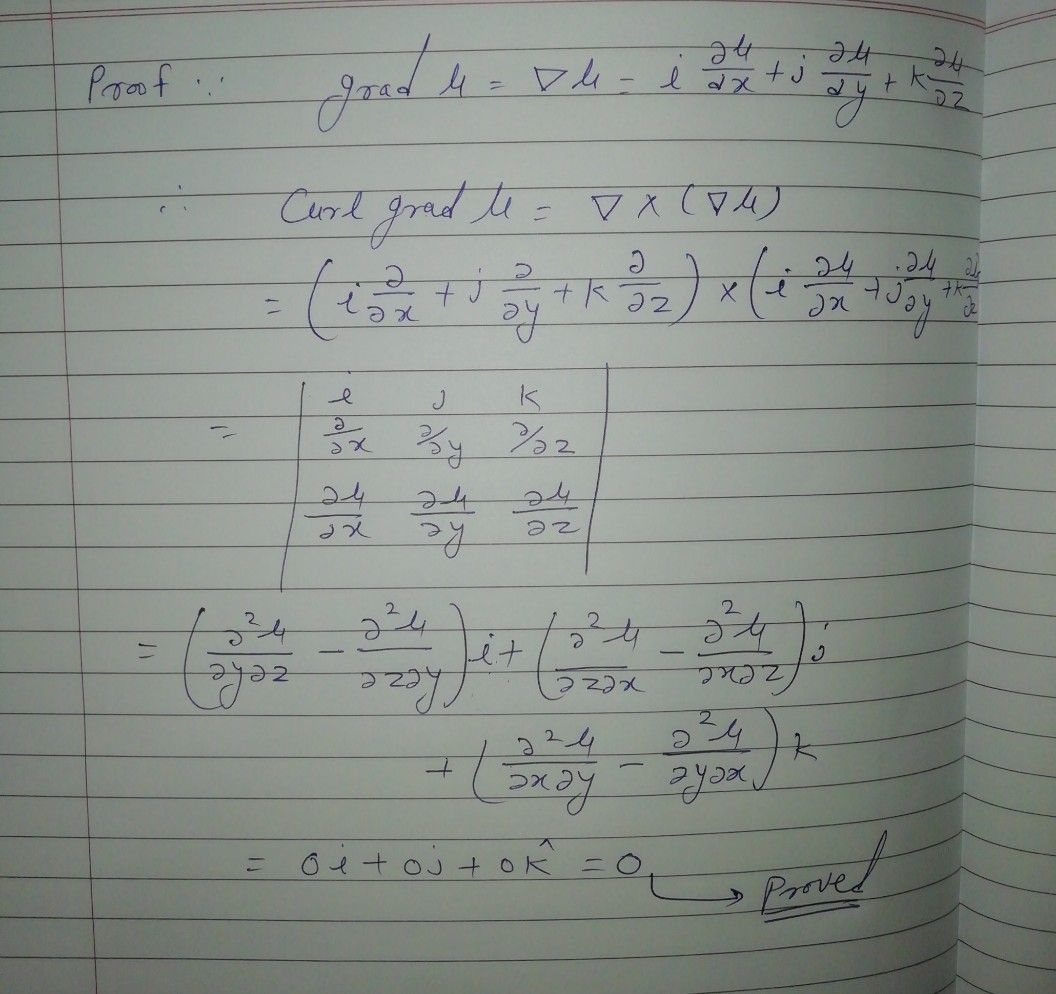Symbol
ProblemProperty $2:$ curl grad $u=0=V\times \left(V4\right)$ i.e. the curl of the gradient of $a$ $sca1a1$ function $7θ$ is $2$ zero. $l$
Other
Search count: 109
SolutionQanda teacher - Aradhyaa